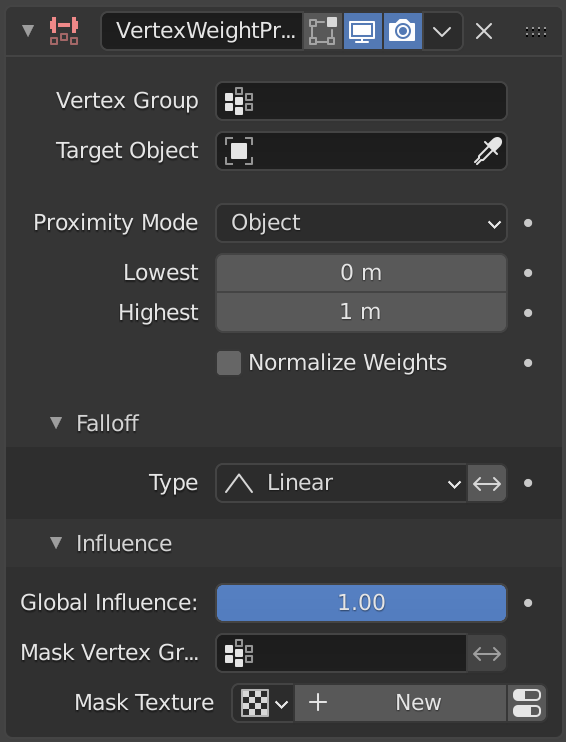# Vertex Weight Proximity Modifier¶

This modifier sets the weights of the given vertex group, based on the distance between the object (or its vertices), and another target object (or its geometry).

Предупреждение

This modifier does implicit clamping of weight values in the standard (0.0 to 1.0) range. All values below 0.0 will be set to 0.0, and all values above 1.0 will be set to 1.0.

Примечание

You can view the modified weights in Weight Paint Mode. This also implies that you will have to disable the Vertex Weight Proximity modifier if you want to see the original weights of the vertex group you are editing.

## Options¶The Vertex Weight Proximity modifier panel.

Vertex Group

The vertex group to affect.

Target Object

The object from which to compute distances.

Proximity mode
Object Distance

Use the distance between the modified mesh object and the target object as weight for all vertices in the affected vertex group.

Geometry Distance

Use the distance between each vertex and the target object, or its geometry.

Vertex

This will set each vertex’s weight from its distance to the nearest vertex of the target object.

Edge

This will set each vertex’s weight from its distance to the nearest edge of the target object.

Face

This will set each vertex’s weight from its distance to the nearest face of the target object.

Примечание

If you enable more than one of them, the shortest distance will be used. If the target object has no geometry (e.g. an empty or camera), it will use the location of the object itself.

Lowest

Distance mapping to 0.0 weight.

Highest

Distance mapping to 1.0 weight.

Совет

Lowest can be set above Highest to reverse the mapping.

Falloff
Falloff Type

Type of mapping.

Linear

No mapping.

Custom Curve

Allows the user to manually define the mapping using a curve.

Sharp, Smooth, Root and Sphere

These are classical mapping functions, from spikiest to roundest.

Random

Uses a random value for each vertex.

Median Step

Creates binary weights (0.0 or 1.0), with 0.5 as cutting value.

Invert <-->

Inverts the falloff.